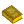## Numerical Integration

The approximate computation of an Integral. The numerical computation of an Integral is sometimes called Quadrature. There are a wide range of methods available for numerical integration. A good source for such techniques is Press et al. (1992).

The most straightforward numerical integration technique uses the Newton-Cotes Formulas (also called Quadrature Formulas), which approximate a function tabulated at a sequent of regularly spaced Intervals by various degree Polynomials. If the endpoints are tabulated, then the 2- and 3-point formulas are called the Trapezoidal Rule and Simpson's Rule, respectively. The 5-point formula is called Bode's Rule. A generalization of the Trapezoidal Rule is Romberg Integration, which can yield accurate results for many fewer function evaluations.

If the functions are known analytically instead of being tabulated at equally spaced intervals, the best numerical method of integration is called Gaussian Quadrature. By picking the abscissas at which to evaluate the function, Gaussian quadrature produces the most accurate approximations possible. However, given the speed of modern computers, the additional complication of the Gaussian Quadrature formalism often makes it less desirable than simply brute-force calculating twice as many points on a regular grid (which also permits the already computed values of the function to be re-used). An excellent reference for Gaussian Quadrature is Hildebrand (1956).

ReferencesNumerical Methods

Davis, P. J. and Rabinowitz, P. Methods of Numerical Integration, 2nd ed. New York: Academic Press, 1984.

Hildebrand, F. B. Introduction to Numerical Analysis. New York: McGraw-Hill, pp. 319-323, 1956.

Milne, W. E. Numerical Calculus: Approximations, Interpolation, Finite Differences, Numerical Integration and Curve Fitting. Princeton, NJ: Princeton University Press, 1949.

Press, W. H.; Flannery, B. P.; Teukolsky, S. A.; and Vetterling, W. T. Numerical Recipes in FORTRAN: The Art of Scientific Computing, 2nd ed. Cambridge, England: Cambridge University Press, 1992.